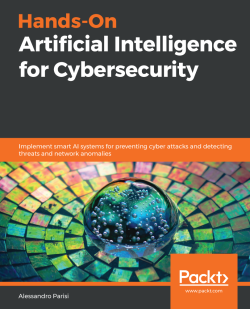### Hands-On Artificial Intelligence for Cybersecurity5 (1 reviews total)
By Alessandro Parisi
• Constantly updated with 100+ new titles each month
• Breadth and depth in over 1,000+ technologies

Today's organizations spend billions of dollars globally on cybersecurity. Artificial intelligence has emerged as a great solution for building smarter and safer security systems that allow you to predict and detect suspicious network activity, such as phishing or unauthorized intrusions.

This cybersecurity book presents and demonstrates popular and successful AI approaches and models that you can adapt to detect potential attacks and protect your corporate systems. You'll learn about the role of machine learning and neural networks, as well as deep learning in cybersecurity, and you'll also learn how you can infuse AI capabilities into building smart defensive mechanisms. As you advance, you'll be able to apply these strategies across a variety of applications, including spam filters, network intrusion detection, botnet detection, and secure authentication.

By the end of this book, you'll be ready to develop intelligent systems that can detect unusual and suspicious patterns and attacks, thereby developing strong network security defenses using AI.

Publication date:
August 2019
Publisher
Packt
Pages
342
ISBN
9781789804027

# Introduction to AI for Cybersecurity Professionals

In this chapter, we'll distinguish between the various branches of Artificial Intelligence (AI), focusing on the pros and cons of the different approaches of automated learning in the field of cybersecurity.

We will introduce different strategies for learning and optimizing of the various algorithms, and we'll also look at the main concepts of AI in action using Jupyter Notebooks and the scikit-learn Python library.

This chapter will cover the following topics:

• Applying AI in cybersecurity
• The evolution from expert systems to data mining and AI
• The different forms of automated learning
• The characteristics of algorithm training and optimization
• Beginning with AI via Jupyter Notebooks
• Introducing AI in the context of cybersecurity

# Applying AI in cybersecurity

The application of AI to cybersecurity is an experimental research area that's not without problems, which we will try to explain during this chapter. However, it is undeniable that the results achieved so far are promising, and that in the near future the methods of analysis will become common practice, with clear and positive consequences in the cybersecurity professional field, both in terms of new job opportunities and new challenges.

When dealing with the topic of applying AI to cybersecurity, the reactions from insiders are often ambivalent. In fact, reactions of skepticism alternate with conservative attitudes, partly caused by the fear that machines will supplant human operators, despite the high technical and professional skills of humans, acquired from years of hard work.

However, in the near future, companies and organizations will increasingly need to invest in automated analysis tools that enable a rapid and adequate response to current and future cybersecurity challenges. Therefore, the scenario that is looming is actually a combination of skills, rather than a clash between human operators and machines. It is therefore likely that the AI within the field of cybersecurity will take charge of the dirty work, that is, the selection of potential suspect cases, leaving the most advanced tasks to the security analysts, letting them investigate in more depth the threats that deserve the most attention.

# Evolution in AI: from expert systems to data mining

To understand the advantages associated with the adoption of AI in the field of cybersecurity, it is necessary to introduce the underlying logic to the different methodological approaches that characterize AI.

We will start with a brief historical analysis of the evolution of AI in order to fully evaluate the potential benefits of applying it in the field of cybersecurity.

# A brief introduction to expert systems

One of the first attempts at automated learning consisted of defining the rule-based decision system applied to a given application domain, covering all the possible ramifications and concrete cases that could be found in the real world. In this way, all the possible options were hardcoded within the automated learning solutions, and were verified by experts in the field.

The fundamental limitation of such expert systems consisted of the fact that they reduced the decisions to Boolean values (which reduce everything down to a binary choice), thus limiting the ability to adapt the solutions to the different nuances of real-world use cases.

In fact, expert systems do not learn anything new compared to hardcoded solutions, but limit themselves to looking for the right answer within a (potentially very large) knowledge base that is not able to adapt to new problems that were not addressed previously.

# Reflecting the indeterministic nature of reality

Since the concrete cases that we come across in the real world cannot simply be represented using just true/false classification models (although experts in the sector strive to list all possible cases, there is always something in reality that escapes classification), it is therefore necessary to make the best use of the data at our disposal in order to let latent tendencies and anomalous cases (such as outliers) emerge, making use of statistical and probabilistic models that can more appropriately reflect the indeterministic nature of reality.

# Going beyond statistics toward machine learning

Although the introduction of statistical models broke through the limitations of expert systems, the underlying rigidity of the approach remained, because statistical models, such as rule-based decisions, were in fact established in advance and could not be modified to adapt to new data. For example, one of the most commonly used statistical models is the Gaussian distribution. The statistician could then decide that the data comes from a Gaussian distribution, and try to estimate the parameters that characterize the hypothetical distribution that best describes the data being analyzed, without taking into consideration alternative models.

To overcome these limits, it was therefore necessary to adopt an iterative approach, which allowed the introduction of machine learning (ML) algorithms capable of generalizing the descriptive models starting from the available data, thus autonomously generating its own features, without limiting itself to predefined target functions, but adapting them to the continuous evolution of the algorithm training process.

# Mining data for models

The difference in approach compared to the predefined static models is also reflected in the research field known as data mining.

An adequate definition of the data mining process consists of the discovery of adequate representative models, starting with the data. Also, in this case, instead of adopting pre-established statistical models, we can use ML algorithms based on the training data to identify the most suitable predictive model (this is more true when we are not able to understand the nature of the data at our disposal).

However, the algorithmic approach is not always adequate. When the nature of the data is clear and conforms to known models, there is no advantage in using ML algorithms instead of pre-defined models. The next step, which absorbs and extends the advantages of the previous approaches, adding the ability to manage cases not covered in the training data, leads us to AI.

AI is a wider field of research than ML, which can manage data of a more generic and abstract nature than ML, thus enabling the transfer of common solutions to different types of data without the need for complete retraining. In this way, it is possible, for example, to recognize objects from color images, starting with objects originally obtained from black and white samples.

Therefore, AI is considered as a broad field of research that includes ML. In turn, ML includes deep learning (DL) which is ML method based on artificial neural networks, as shown in the following diagram: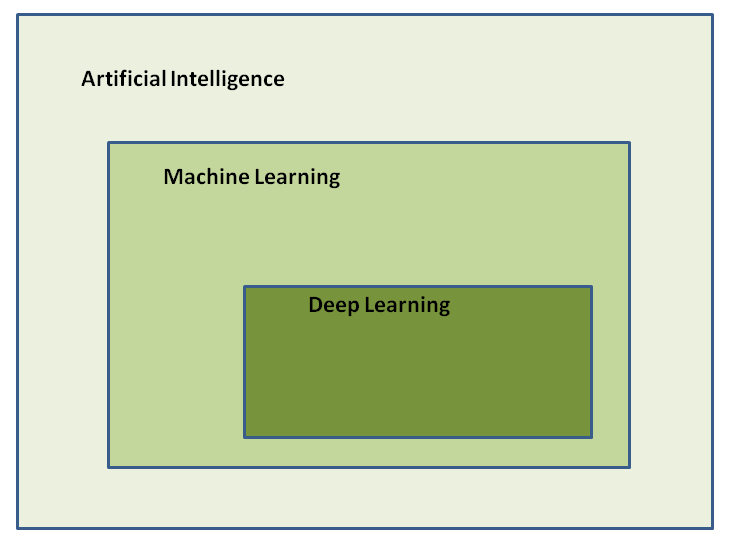# Types of machine learning

The process of mechanical learning from data can take different forms, with different characteristics and predictive abilities.

In the case of ML (which, as we have seen, is a branch of research belonging to AI), it is common to distinguish between the following types of ML:

• Supervised learning
• Unsupervised learning
• Reinforcement learning

The differences between these learning modalities are attributable to the type of result (output) that we intend to achieve, based on the nature of the input required to produce it.

# Supervised learning

In the case of supervised learning, algorithm training is conducted using an input dataset, from which the type of output that we have to obtain is already known.

In practice, the algorithms must be trained to identify the relationships between the variables being trained, trying to optimize the learning parameters on the basis of the target variables (also called labels) that, as mentioned, are already known.

An example of a supervised learning algorithm is classification algorithms, which are particularly used in the field of cybersecurity for spam classification.

A spam filter is in fact trained by submitting an input dataset to the algorithm containing many examples of emails that have already been previously classified as spam (the emails were malicious or unwanted) or ham (the emails were genuine and harmless).

The classification algorithm of the spam filter must therefore learn to classify the new emails it will receive in the future, referring to the spam or ham classes based on the training previously performed on the input dataset of the already classified emails.

Another example of supervised algorithms is regression algorithms. Ultimately, there are the following main supervised algorithms:

• Regression (linear and logistic)
• k-Nearest Neighbors (k-NNs)
• Support vector machines (SVMs)
• Decision trees and random forests
• Neural networks (NNs)

# Unsupervised learning

In the case of unsupervised learning, the algorithms must try to classify the data independently, without the aid of a previous classification provided by the analyst. In the context of cybersecurity, unsupervised learning algorithms are important for identifying new (not previously detected) forms of malware attacks, frauds, and email spamming campaigns.

Here are some examples of unsupervised algorithms:

• Dimensionality reduction:
• Principal component analysis (PCA)
• PCA Kernel
• Clustering:
• k-means
• Hierarchical cluster analysis (HCA)

# Reinforcement learning

In the case of reinforcement learning (RL), a different learning strategy is followed, which emulates the trial and error approach. Thus, drawing information from the feedback obtained during the learning path, with the aim of maximizing the reward finally obtained based on the number of correct decisions that the algorithm has selected.

In practice, the learning process takes place in an unsupervised manner, with the particularity that a positive reward is assigned to each correct decision (and a negative reward for incorrect decisions) taken at each step of the learning path. At the end of the learning process, the decisions of the algorithm are reassessed based on the final reward achieved.

Given its dynamic nature, it is no coincidence that RL is more similar to the general approach adopted by AI than to the common algorithms developed in ML.

The following are some examples of RL algorithms:

• Markov process
• Q-learning
• Temporal difference (TD) methods
• Monte Carlo methods

In particular, Hidden Markov Models (HMM) (which make use of the Markov process) are extremely important in the detection of polymorphic malware threats.

# Algorithm training and optimization

When preparing automated learning procedures, we will often face a series of challenges. We need to overcome these challenges in order to recognize and avoid compromising the reliability of the procedures themselves, thus preventing the possibility of drawing erroneous or hasty conclusions that, in the context of cybersecurity, can have devastating consequences.

One of the main problems that we often face, especially in the case of the configuration of threat detection procedures, is the management of false positives; that is, cases detected by the algorithm and classified as potential threats, which in reality are not. We will discuss false positives and ML evaluation metrics in more depth in Chapter 7Fraud Prevention with Cloud AI Solutions, and Chapter 9, Evaluating Algorithms.

The management of false positives is particularly burdensome in the case of detection systems aimed at contrasting networking threats, given that the number of events detected are often so high that they absorb and saturate all the human resources dedicated to threat detection activities.

On the other hand, even correct (true positive) reports, if in excessive numbers, contribute to functionally overloading the analysts, distracting them from priority tasks. The need to optimize the learning procedures therefore emerges in order to reduce the number of cases that need to be analyzed in depth by the analysts.

This optimization activity often starts with the selection and cleaning of the data submitted to the algorithms.

# How to find useful sources of data

In the case of anomaly detection, for example, particular attention must be paid to the data being analyzed. An effective anomaly detection activity presupposes that the training data does not contain the anomalies sought, but that on the contrary, they reflect the normal situation of reference.

If, on the other hand, the training data was biased with the anomalies being investigated, the anomaly detection activity would lose much of its reliability and utility in accordance with the principle commonly known as GIGO, which stands for garbage in, garbage out.

Given the increasing availability of raw data in real time, often the preliminary cleaning of data is considered a challenge in itself. In fact, it's often necessary to conduct a preliminary skim of the data, eliminating irrelevant or redundant information. We can then present the data to the algorithms in a correct form, which can improve their ability to learn, adapting to the form of data on the basis of the type of algorithm used.

For example, a classification algorithm will be able to identify a more representative and more effective model in cases in which the input data will be presented in a grouped form, or is capable of being linearly separable. In the same way, the presence of variables (also known as dimensions) containing empty fields weighs down the computational effort of the algorithm and produces less reliable predictive models due to the phenomenon known as the curse of dimensionality.

This occurs when the number of features, that is, dimensions, increases without improving the relevant information, simply resulting in data being dispersed in the increased space of research:Also, the sources from which we draw our test cases (samples) are important. Think, for example, of a case in which we have to predict the mischievous behavior of an unknown executable. The problem in question is reduced to the definition of a model of classification of the executable, which must be traced back to one of two categories: genuine and malicious.

To achieve such a result, we need to train our classification algorithm by providing it with a number of examples of executables that are considered malicious as an input dataset.

# Quantity versus quality

When it all boils down to quantity versus quality, we are immediately faced with the following two problems:

• What types of malware can we consider most representative of the most probable risks and threats to our company?
• How many example cases (samples) should we collect and administer to the algorithms in order to obtain a reliable result in terms of both effectiveness and predictive efficiency of future threats?

The answers to the two questions are closely related to the knowledge that the analyst has of the specific organizational realm in which they must operate.

All this could lead the analyst to believe that the creation of a honey-pot, which is useful for gathering malicious samples in the wild that will be fed to the algorithms as training samples, would be more representative of the level of risk to which the organization is exposed than the use of datasets as examples of generic threats. At the same time, the number of test examples to be submitted to the algorithm is determined by the characteristics of the data themselves. These can, in fact, present a prevalence of cases (skewness) of a certain type, to the detriment of other types, leading to a distortion in the predictions of the algorithm toward the classes that are most numerous, when in reality, the most relevant information for our investigation is represented by a class with a smaller number of cases.

In conclusion, it will not be a matter of being able to simply choose the best algorithm for our goals (which often does not exist), but mainly to select the most representative cases (samples) to be submitted to a set of algorithms, which we will try to optimize based on the results obtained.

# Getting to know Python's libraries

In the following sections, we will explore the concepts presented so far, presenting some sample code that make use of a series of Python libraries that are among the most well known and widespread in the field of ML:

• NumPy (version 1.13.3)
• pandas (version 0.20.3)
• Matplotlib (version 2.0.2)
• scikit-learn (version 0.20.0)
• Seaborn (version 0.8.0)

The sample code will be shown here in the form of snippets, along with screenshots representing their output. Do not worry if not all of the implementation details are clear to you at first glance; we will have the opportunity to understand the implementation aspects of every single algorithm throughout the book.

# Supervised learning example – linear regression

As our first example, we'll look at one of the most commonly used algorithms in the field of supervised learning, namely linear regression. Taking advantage of the scikit-learn Python library, we instantiate a linear regression object, by importing the LinearRegression class included in the linear_model package of the scikit-learn library.

The model will be trained with a training dataset obtained by invoking the rand() method of the RandomState class, which belongs to the random package of the Python numpy library. The training data is distributed following the linear model of,. The training of the model is carried out by invoking the fit() method on the lreg object of the LinearRegression class.

At this point, we will try to predict data that is not included in the training dataset by invoking the predict() method on the lreg object.

The training dataset, together with the values interpolated by the model, are finally printed on screen using the scatter() and plot() methods of the matplotlib library:

```%matplotlib inlineimport matplotlib.pyplot as pltimport numpy as np
from sklearn.linear_model import LinearRegression
pool = np.random.RandomState(10)x = 5 * pool.rand(30)y = 3 * x - 2 + pool.randn(30)# y = 3x - 2;
lregr = LinearRegression(fit_intercept=False)
X = x[:, np.newaxis]lregr.fit(X, y)lspace = np.linspace(0, 5)X_regr = lspace[:, np.newaxis]y_regr = lregr.predict(X_regr)plt.scatter(x, y);plt.plot(X_regr, y_regr);```

The preceding code generates the following output, which shows how well the data samples are approximated by the straight line returned by the LinearRegression model: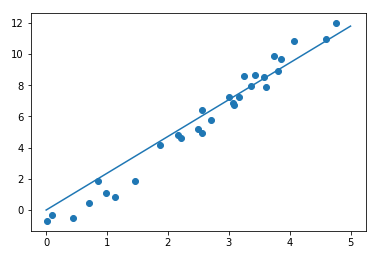# Unsupervised learning example – clustering

As an example of unsupervised learning, we use the GaussianMixture clustering model. Through this type of model, we will try to bring the data back to a collection of Gaussian blobs.

The training data is loaded from a file in .csv format (comma-separated values) and stored in a DataFrame object of the pandas Python library. Once the data is loaded, we proceed to reduce its dimensionality in order to identify a representation that reduces the original dimensions (features) from four to two, trying to maintain the features that are most representative of the samples.

The reduction of dimensionality prevents the disadvantages connected to the phenomenon of the curse of dimensionality, improves the computational efficiency, and simplifies the visualization of the data.

The technique we will use for dimensionality reduction is known as principal component analysis (PCA), and is available in the scikit-learn library.

Once the data dimensions are reduced from four to two, we will try to classify the data using the GaussianMixture model as follows:

```import pandas as pd
import seaborn as sns
data_df.describe()
X_data = data_df.drop('class_1', axis=1)
y_data = data_df['class_1']

from sklearn.decomposition import PCA
pca = PCA(n_components=2)
pca.fit(X_data)
X_2D = pca.transform(X_data)
data_df['PCA1'] = X_2D[:, 0]
data_df['PCA2'] = X_2D[:, 1]

from sklearn.mixture import GaussianMixture
gm = GaussianMixture(n_components=3, covariance_type='full')
gm.fit(X_data)                         y_gm = gm.predict(X_data)
data_df['cluster'] = y_gm
sns.lmplot("PCA1", "PCA2", data=data_df, col='cluster', fit_reg=False)```

As can be seen in the following screenshot, the clustering algorithm has succeeded in classifying the data automatically in an appropriate manner, without having previously received information on the current labels associated with the various samples: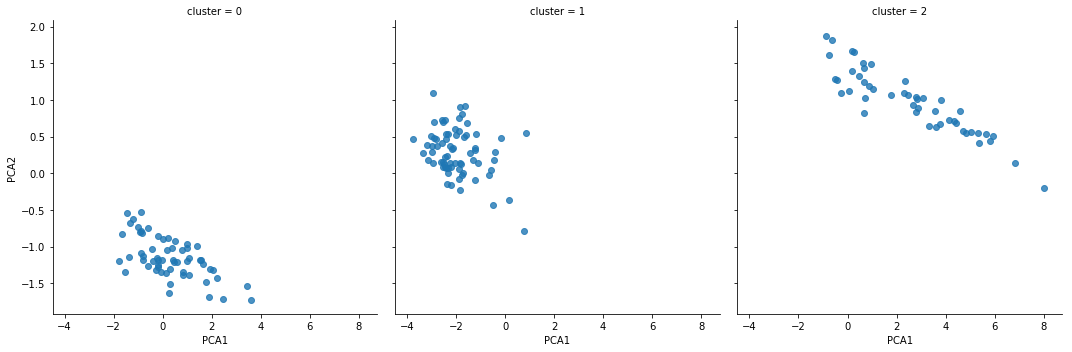# Simple NN example – perceptron

In this section, we will show a simple NN model, known as a perceptron.

NNs and DL are subfields of ML aimed at emulating the human brain's learning capabilities. NN and DL will be addressed in more depth in Chapter 3Ham or Spam? Detecting Email Cybersecurity Threats with AI, and Chapter 8, GANs – Attacks and Defenses.

However rudimentary it is, a perceptron is nonetheless able to adequately classify samples that tend to group together (in technical terms, those that are linearly separable).

One of the most common uses of a perceptron in the field of cybersecurity, as we will see, is in the area of spam filtering.

In the following example, we will use the scikit-learn implementation of the perceptron algorithm:

`from matplotlib.colors import ListedColormap # Thanks to Sebastian Raschka for 'plot_decision_regions' function def plot_decision_regions(X, y, classifier, resolution=0.02):  # setup marker generator and color map  markers = ('s', 'x', 'o', '^', 'v') colors = ('red', 'blue', 'lightgreen', 'gray', 'cyan') cmap = ListedColormap(colors[:len(np.unique(y))])  # plot the decision surface  x1_min, x1_max = X[:, 0].min() - 1, X[:, 0].max() + 1 x2_min, x2_max = X[:, 1].min() - 1, X[:, 1].max() + 1 xx1, xx2 = np.meshgrid(np.arange(x1_min, x1_max, resolution),  np.arange(x2_min, x2_max, resolution)) Z = classifier.predict(np.array([xx1.ravel(), xx2.ravel()]).T) Z = Z.reshape(xx1.shape) plt.contourf(xx1, xx2, Z, alpha=0.4, cmap=cmap) plt.xlim(xx1.min(), xx1.max()) plt.ylim(xx2.min(), xx2.max())  # plot class samples  for idx, cl in enumerate(np.unique(y)): plt.scatter(x=X[y == cl, 0], y=X[y == cl, 1],  alpha=0.8, c=cmap(idx),  marker=markers[idx], label=cl) from sklearn.linear_model import perceptronfrom sklearn.datasets import make_classification X, y = make_classification(30, 2, 2, 0, weights=[.3, .3], random_state=300) plt.scatter(X[:,0], X[:,1], s=50)pct = perceptron.Perceptron(max_iter=100, verbose=0, random_state=300, fit_intercept=True, eta0=0.002)pct.fit(X, y)plot_decision_regions(X, y, classifier=pct)plt.title('Perceptron')plt.xlabel('X')plt.ylabel('Y')plt.show()`

The preceding code generates the following output: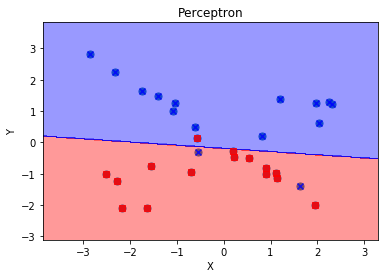# AI in the context of cybersecurity

With the exponential increase in the spread of threats associated with the daily diffusion of new malware, it is practically impossible to think of dealing effectively with these threats using only analysis conducted by human operators. It is necessary to introduce algorithms that allow us to automate that introductory phase of analysis known as triage, that is to say, to conduct a preliminary screening of the threats to be submitted to the attention of the cybersecurity professionals, allowing us to respond in a timely and effective manner to ongoing attacks.

We need to be able to respond in a dynamic fashion, adapting to the changes in the context related to the presence of unprecedented threats. This implies not only that the analysts manage the tools and methods of cybersecurity, but that they can also correctly interpret and evaluate the results offered by AI and ML algorithms.

Cybersecurity professionals are therefore called to understand the logic of the algorithms, thus proceeding to the fine tuning of their learning phases, based on the results and objectives to be achieved.

Some of the tasks related to the use of AI are as follows:

• Classification: This is one of the main tasks in the framework of cybersecurity. It's used to properly identify types of similar attacks, such as different pieces of malware belonging to the same family, that is, having common characteristics and behavior, even if their signatures are distinct (just think of polymorphic malware). In the same way, it is important to be able to adequately classify emails, distinguishing spam from legitimate emails.
• Clustering: Clustering is distinguished from classification by the ability to automatically identify the classes to which the samples belong when information about classes is not available in advance (this is a typical goal, as we have seen, of unsupervised learning). This task is of fundamental importance in malware analysis and forensic analysis.
• Predictive analysis: By exploiting NNs and DL, it is possible to identify threats as they occur. To this end, a highly dynamic approach must be adopted, which allows algorithms to optimize their learning capabilities automatically.

Possible uses of AI in cybersecurity are as follows:

• Network protection: The use of ML allows the implementation of highly sophisticated intrusion detection systems (IDS), which are to be used in the network perimeter protection area.
• Endpoint protection: Threats such as ransomware can be adequately detected by adopting algorithms that learn the behaviors that are typical of these types of malware, thus overcoming the limitations of traditional antivirus software.
• Application security: Some of the most insidious types of attacks on web applications include Server Side Request Forgery (SSRF) attacks, SQL injection, Cross-Site Scripting (XSS), and Distributed Denial of Service (DDoS) attacks. These are all types of threats that can be adequately countered by using AI and ML tools and algorithms.
• Suspect user behavior: Identifying attempts at fraud or compromising applications by malicious users at the very moment they occur is one of the emerging areas of application of DL.

# Summary

In this chapter, we have introduced the fundamental concepts of AI and ML in relation to the context of cybersecurity. We have presented some of the strategies adopted in the management of automated learning process, and the possible problems that data analysts face. The concepts and tools that we have learned in this chapter will be used and adapted in the following chapters, addressing the specific problems of cybersecurity.

In the next chapter, we will learn how to manage Jupyter interactive notebooks in more depth, which allows the reader to interactively execute the instructions given and display the results of the execution in real time.

During the course of the book, the concepts of AI and ML will be presented from time to time in the topics covered in the individual chapters, trying to provide a practical interpretation of the algorithms examined. For those interested in examining the implementation details of the various algorithms used, we suggest that you consult Python Machine Learning - Second Edition by Sebastian Raschka and Vahid Mirjalili, published by Packt Publishing.